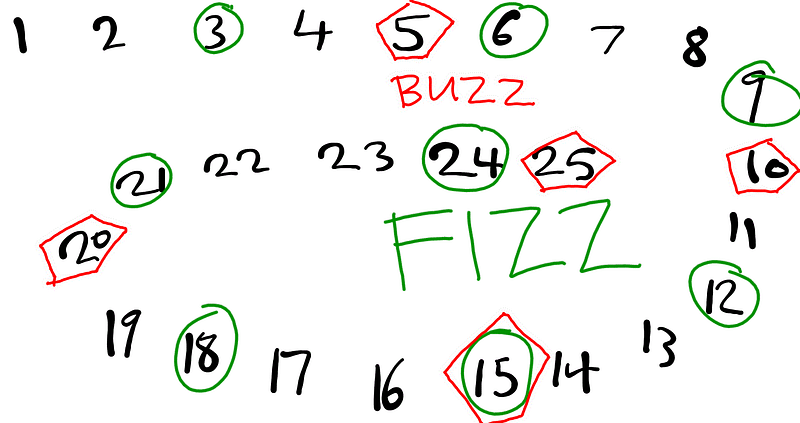# JavaScript Algorithms Part 3. FIZZBUZZImage Credits: GetYourImage.club

The FizzBuzz algorithm question is one very popular algorithm problem lots of folks would have to solve, in fact, it is a very popular interview question for programmers.

Question.

Write a program that prints the numbers from 1 to n. But for multiples of three print “Fizz” instead of the number and for the multiples of five print “Buzz”. For numbers which are multiples of both three and five print “FizzBuzz”

So we would be writing a function that prints from 1 to let’s say 15. We would print ‘Fizz’ for multiples of 3, ‘Buzz’ for multiples of 5 and ‘FizzBuzz’ for multiples of 3 and 5.
So if n = 15, we expect the results to be:

1, 2, Fizz, 4, Buzz,
Fizz, 7, 8, Fizz, Buzz,
11, Fizz, 13, 14, FizzBuzz


To solve this problem, we need to know about the modulo operator in JavaScript and how it works. The modulo operator is represented as % in JavaScript.

What is the modulo operator?
This operator returns the remainder after performing mathematical division on a number eg:

let numberOne = 9 % 5;
console.log(numberOne);
// returns 4

let numberTwo = 9 % 3;
console.log(numberTwo);
// returns 0

let numberThree = 9 % 2;
console.log(numberThree);
// returns 1


From the above, we can deduce that if the result of calling the modulo operator on a number n is 0, it is safe to say that n is divisible by the number.

This means if n % 3 = 0 that is a Fizz, if n % 5 = 0 that is a Buzz, and if n % 3 = 0 && n % 5 = 0, then that is a FizzBuzz.

So to solve this problem, we would simply loop through from number 1 to a given number n and return the appropriate strings we want to be printed out like so:

const fizzBuzz = n => {

(let i = 1; i <= n; i++) {

(i % 3 === 0 && i % 5 === 0){
console.log('fizzbuzz')
}

( i % 3 === 0){
console.log('fizz')
}

(i % 5 === 0){
console.log('buzz')
}

{
console.log(i)
}
}
}


The code snippet below will return fizzbuzz for numbers divisible by 3 and 5:

 (i % 3 === 0 && i % 5 === 0){
console.log('fizzbuzz')
}


The code snippet below would return fizz for numbers divisible by 3:

 (i % 3 === 0){
console.log('fizz')
}


The code snippet below would return buzz for numbers divisible by 5:

 (i % 5=== 0){
console.log('buzzzz')
}


Then, the last code snippet would return every other number within the loop not divisible by 3 or 5.

console.log(i)


To try out your own approach to solving this problem.
- Clone https://github.com/seunzone/js-algorithms
- Install dependencies using yarn install (Assumes you have node installed on your computer).
- Write your code in the index.js file of the fizzbuzz folder.
- In the terminal run yarn run fizzbuzz to see if your code passes the test.

Thanks for reading.